# Image Matching Techniques

## Producer Field Guide

HGD_Product
Producer Field Guide
HGD_Portfolio_Suite
Producer

Image matching refers to the automatic identification and measurement of corresponding image points that are located on the overlapping area of multiple images. The various image matching methods can be divided into three categories including:

• Area based matching
• Feature based matching
• Relation based matching

Area Based Matching

Area based matching is also called signal based matching. This method determines the correspondence between two image areas according to the similarity of their gray level values. The cross correlation and least squares correlation techniques are well-known methods for area based matching.

Correlation Windows

Area based matching uses correlation windows. These windows consist of a local neighborhood of pixels. One example of correlation windows is square neighborhoods (for example, 3 × 3, 5 × 5, 7 × 7 pixels). In practice, the windows vary in shape and dimension based on the matching technique. Area correlation uses the characteristics of these windows to match ground feature locations in one image to ground features on the other.

A reference window is the source window on the first image, which remains at a constant location. Its dimensions are usually square in size (for example, 3 × 3, 5 × 5, and so on). Search windows are candidate windows on the second image that are evaluated relative to the reference window. During correlation, many different search windows are examined until a location is found that best matches the reference window.

Correlation Calculations

Two correlation calculations are described below: cross correlation and least squares correlation. Most area based matching calculations, including these methods, normalize the correlation windows. Therefore, it is not necessary to balance the contrast or brightness prior to running correlation. Cross correlation is more robust in that it requires a less accurate a priori position than least squares. However, its precision is limited to one pixel. Least squares correlation can achieve precision levels of one-tenth of a pixel, but requires an a priori position that is accurate to about two pixels. In practice, cross correlation is often followed by least squares for high accuracy.

Cross Correlation

Cross correlation computes the correlation coefficient of the gray values between the template window and the search window according to the following equation: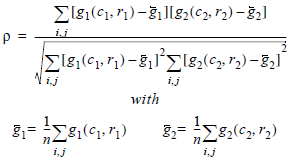Where: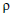= correlation coefficient

g(c,r) = gray value of the pixel (c,r)

c1, r1 = pixel coordinates on the left image

c2, r2 = pixel coordinates on the right image

n = total number of pixels in the window

i, j = pixel index into the correlation window

When using the area based cross correlation, it is necessary to have a good initial position for the two correlation windows. If the exterior orientation parameters of the images being matched are known, a good initial position can be determined. Also, if the contrast in the windows is very poor, the correlation can fail.

Least Squares Correlation

Least squares correlation uses the least squares estimation to derive parameters that best fit a search window to a reference window. This technique has been investigated thoroughly in photogrammetry (Ackermann, 1983; Grün and Baltsavias, 1988; Helava, 1988). It accounts for both gray scale and geometric differences, making it especially useful when ground features on one image look somewhat different on the other image (differences which occur when the surface terrain is quite steep or when the viewing angles are quite different).

Least squares correlation is iterative. The parameters calculated during the initial pass are used in the calculation of the second pass and so on, until an optimum solution is determined. Least squares matching can result in high positional accuracy (about 0.1 pixels). However, it is sensitive to initial approximations. The initial coordinates for the search window prior to correlation must be accurate to about two pixels or better.

When least squares correlation fits a search window to the reference window, both radiometric (pixel gray values) and geometric (location, size, and shape of the search window) transformations are calculated.

For example, suppose the change in gray values between two correlation windows is represented as a linear relationship. Also assume that the change in the window’s geometry is represented by an affine transformation.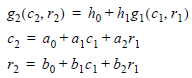Where:

c1,r1 = pixel coordinate in the reference window

c2,r2 = pixel coordinate in the search window

g1(c1r1) = gray value of pixel (c1,r1)

g2(c2,r2) = gray value of pixel (c1,r1)

h0, h1 = linear gray value transformation parameters

a0, a1, a2 = affine geometric transformation parameters

b0, b1, b2 = affine geometric transformation parameters

Based on this assumption, the error equation for each pixel is derived, as shown in the following equation: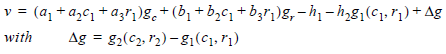Where:

gc and gr are the gradients of g2 (c2,r2).

Feature Based Matching

Feature based matching determines the correspondence between two image features. Most feature based techniques match extracted point features (this is called feature point matching), as opposed to other features, such as lines or complex objects. The feature points are also commonly referred to as interest points. Poor contrast areas can be avoided with feature based matching.

In order to implement feature based matching, the image features must initially be extracted. There are several well-known operators for feature point extraction. Examples include the Moravec Operator, the Dreschler Operator, and the Förstner Operator (Förstner and Gülch, 1987; Lü, 1988).

After the features are extracted, the attributes of the features are compared between two images. The feature pair having the attributes with the best fit is recognized as a match. IMAGINE Photogrammetry Project Manager uses the Förstner interest operator to extract feature points.

Relation Based Matching

Relation based matching is also called structural matching (Vosselman and Haala, 1992; Wang, Y., 1994; and Wang, Y., 1995). This kind of matching technique uses the image features and the relationship between the features. With relation based matching, the corresponding image structures can be recognized automatically, without any a priori information. However, the process is time-consuming since it deals with varying types of information. Relation based matching can also be applied for the automatic recognition of control points.

Image Pyramid

Because of the large amount of image data, the image pyramid is usually adopted during the image matching techniques to reduce the computation time and to increase the matching reliability. The pyramid is a data structure consisting of the same image represented several times, at a decreasing spatial resolution each time. Each level of the pyramid contains the image at a particular resolution.

The matching process is performed at each level of resolution. The search is first performed at the lowest resolution level and subsequently at each higher level of resolution. The following figure shows a four-level image pyramid.

Image Pyramid for Matching at Coarse to Full Resolution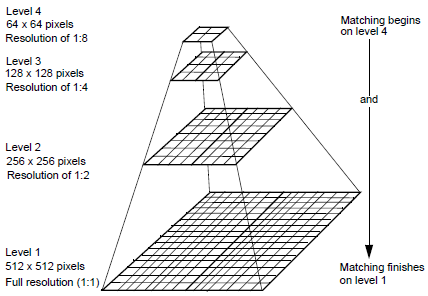There are different resampling methods available for generating an image pyramid. Theoretical and practical investigations show that the resampling methods based on the Gaussian filter, which are approximated by a binomial filter, have the superior properties concerning preserving the image contents and reducing the computation time (Wang, Y., 1994).

The Compute Pyramid Layers option in IMAGINE has three options for continuous image data (raster images); 2x2, 3x3, or 4x4 kernel size filtering methods. The 3x3 option, known as Binomial Interpolation, is strongly recommended for IMAGINE Photogrammetry and Stereo Analyst modules.

Binomial Interpolation uses calculations of 9 pixels in a 3x3 pixel window of the higher resolution level and applies the result to one pixel for the current level of pyramid. As noted, resampling methods based on the Gaussian filter have superior properties concerning preserving the image contents and reduction of computation time, however, the Gaussian filter method is sophisticated and very time-consuming. Therefore in practice, binomial filters are used to approximate the Gaussian filter. The 3x3 binomial filter can be represented as: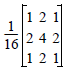An advantage of the Binomial filter is fast and simple computation, because a two-dimensional binomial filter can be decomposed to two one-dimensional filters and finally to simple addition operations and shift.

For some photogrammetric processes such as automatic tie point collection and automatic DEM extraction, the pyramid layers will be used to reduce computation time and increase reliability. These processes require that the pyramid layers fade out the detailed features, but retain the main features. The binomial filter meets this requirement, producing a moderate degree of image smoothing.

In contrast, while the 2x2 kernel filter produces good results for image visual observation, it can smooth or sharpen the image considerably, causing more detail to be lost than desired.

The Binomial Interpolation method preserves the original image information more effectively since the nature of optical imaging and resampling theory are considered in the calculations. Detailed image features are gradually faded out while significant image features are retained, meeting requirements for fast and reliable image matching processes. The computation speed for this method is similar to 2x2 kernel.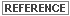See Pyramid Layers for a description of 2x2 and 4x4 kernel sizes.